## Average For SBI PO set 3

1. The average of 5 numbers is 306.4. The average of the first two numbers is 431 and the average of the last two numbers is 214.5. What is the third number?

(a) 108

(b) 52

(c) 321

(d) Cannot be determined

(e) None of these

(e) None of these

Explanation:

According to the question, sum of five numbers = 5 × 306.4 = 1532

Third number = 1532 -2×431 – 2 × 214.5

= 1532 – 862 – 429 = 241

2. Kamlesh bought 65 books for ₹ 1, 050 from one shop and 50 books ₹ 1, 020 from another. What is the average price he paid per book?

(a) ₹ 36.40

(b₹ 18.20

(c) ₹ 24

(d) ₹ 18

(e) None of these

(d) ₹ 18

Explanation: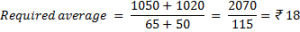3. The sum of five numbers is 290. The average of the first two numbers is 48.5 and the average of last two numbers is 53.5. What is the third number?

(a) 72

(b) 84

(c) 96

(d) 108

(e) None of these

(e) None of these

Explanation:

Third number = 290 – 2× 48.5 – 2 × 53.5

= 290 – 97 – 107 = 86

4. The average weight of a group of 53 girls was calculated as 58 kg. It was later discovered that the weight of one of the girls was read as 65 kg. Whereas her actual weight was 45 kg. What is the actual average weight of the group of 53 girls? (rounded off to two digits after decimal)

(a) 58.62 kg

(b) 58.37 kg

(c) 57.37 kg

(d) 57.62 kg

(e) None of these

(d) 57.62 kg

Explanation:

Actual weight of 53 girls = 53 × 58 + 45 – 65

= 3074 + 45 – 65 = 3054 kg.

Required actual average weight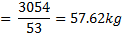Or

Decrease is weight = 65 – 45 = 20 kg

Required actual average weight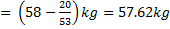5. The average marks in science subject of a class of 20 students is 68. If he marks of two students were misread as 48 and 65 of the actual marks 72 and 61 respectively, then what would be th correct average?

(a) 68.5

(b) 69

(c) 69.5

(d) 70

(e) 66

(b) 69

Explanation:

Difference of marks = 72+61- 48 – 65 = 20

Correct average marks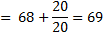6. The average age family of five members is 24. If the present age of youngest member is 8 years, then what was the average age of the family at the time of the birth of the youngest member?

(a) 20 years

(b) 16 years

(c) 12 years

(d) 18 years

(e) 21 years

(b) 16 years

Explanation:

Total age of 5 member family = 24 × 5 = 120 years

Total age 8 years ago = 120- 5 × 8 = 80 years

Required average age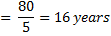7. The average of 5 numbers is 65. The average of the first two numbers last two numbers is 38. What is the third number?

(a) 63

(b) 87

(c) 99

(d) Cannot be determined

(e) None of these

(b) 87

Explanation:

The third number = 5 × 65 -2 × 81 -2 ×38

= 325 – 162 – 76 = 87

8. The total marks obtained by a student in physics, chemistry and mathematics together is 120 more than the marks obtained by him chemistry. What are the average marks obtained by him in physics and mathematics together?

(a) 60

(b) 120

(c) 40

(d) Cannot be determined

(e) None of these

(a) 60

Explanation:

according to question,

P + C + M = 120 +C

P + M = 120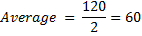9. The average age of 80 boys in a class is 15 years. The average age of a group of 15 boys in the class is 16 years and the average age of another 25 boys in the class is 14 years. What is the average age of the remaining in the class?

(a) 15.25 years

(b) 14 years

(c) 14.75 years

(d) Cannot be determined

(e) None of these

(a) 15.25 years

Explanation:

Let the required average age be x years.

Then 80 × 15 = 15 × 16 + 25 × 14 + 40 × x

40x = 1200 – 240 – 350 = 610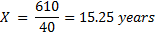10. The average age of seven boys sitting in a row facing north is 26 years. If the average age of first three boys is 19 years and the average age of last three boys is 32 years, what is the age of the boy who is sitting in middle of the row?

(a) 28 years

(b) 29 years

(c) 24 years

(d) 31 years

(e) None of these